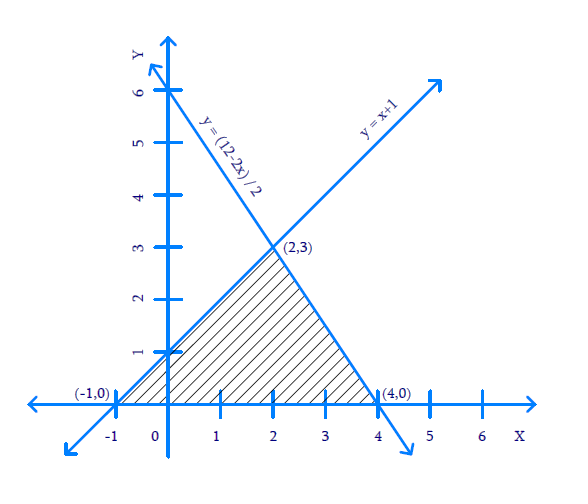# Ex.3.2 Q7 Pair of Linear Equations in Two Variables Solution - NCERT Maths Class 10

Go back to  'Ex.3.2'

## Question

Draw the graphs of the equations $$x-y + 1 = 0$$ and $$3x + 2y-12 = 0.$$ Determine the coordinates of the vertices of the triangle formed by these lines and the $$x-$$axis and shade the triangular region.

Video Solution
Pair Of Linear Equations In Two Variables
Ex 3.2 | Question 7

## Text Solution

What is Known?

Linear equation

\begin{align}x - y + 1 &= 0\\3x + 2y-12 &= 0\end{align}

What is Unknown?

Coordinates of the vertices of the triangle formed by intersecting lines and the $$x$$-axis

Reasoning:

From graph of two linear equations and $$x$$-axis, triangle can be shaded, and vertices can be located.

Steps:

\begin{align}x - y + 1 &= 0\\y &= x + 1\end{align}

 $$x$$ $$0$$ $$1$$ $$y = x + 1$$ $$1$$ $$2$$

\begin{align}3x + 2y-12 &= 0\\2y &= 12 - 3x\\y &= \frac{{12 - 3x}}{2}\end{align}

 $$x$$ $$0$$ $$2$$ $$y = \frac{{12 - 3x}}{2}$$ $$6$$ $$3$$From graph, Vertices are $$(-1, \,0),\; (4,\, 0),$$ and $$(2, \,3)$$

Learn from the best math teachers and top your exams

• Live one on one classroom and doubt clearing
• Practice worksheets in and after class for conceptual clarity
• Personalized curriculum to keep up with school